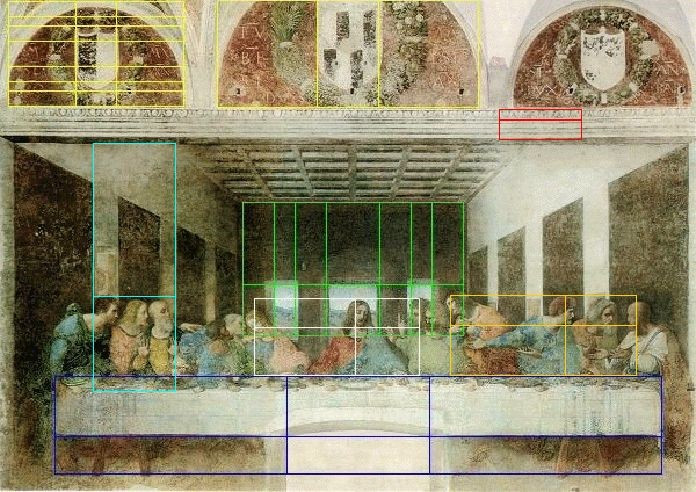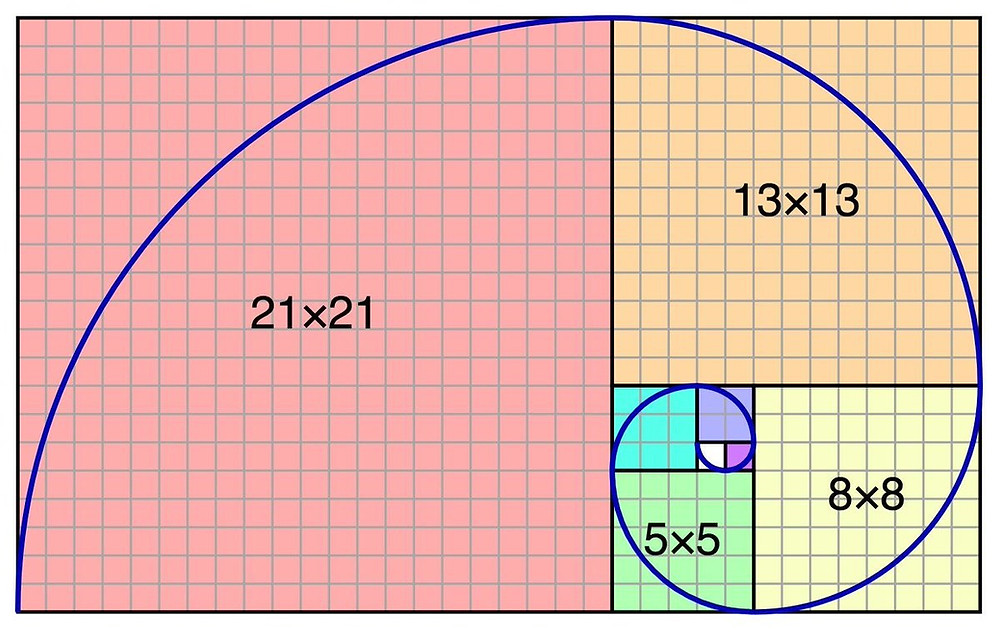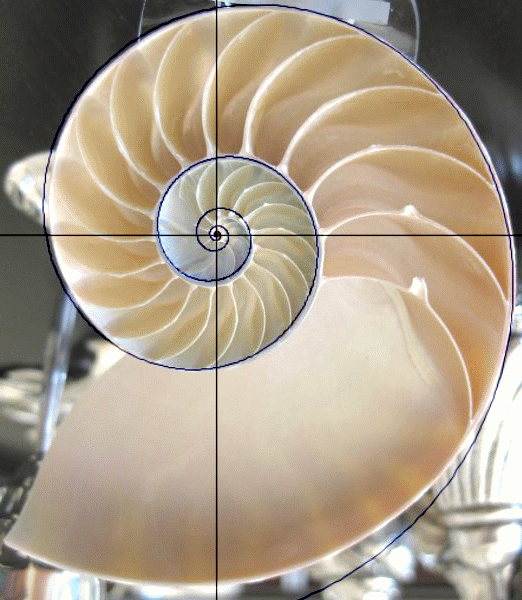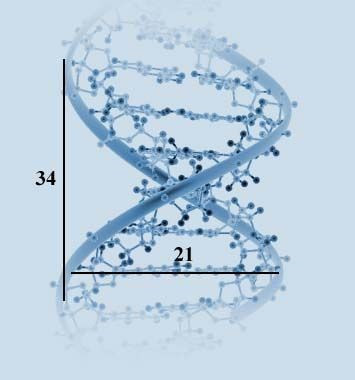Search

# The Fibonacci Phenomenon

Fibonacci forms the basis of some important utensils in many well-equipped technical analysts’ toolboxes, but what is Fibonacci, and where do the ratios come from?

The Fibonacci ratios derive from the Fibonacci sequence of numbers, named after the 13th Century Italian mathematician, Leonardo Fibonacci. He most famously wrote of the Fibonacci sequence when examining the population of rabbits as they breed. He modelled the rabbit population such that after each generation, the population was the sum of the two populations which had preceded it. The study resulted in the following sequence:

1 , 1 , 2 , 3 , 5 , 8 , 13 , 21 , 34 , 55 , 89, . . .

The term-to-term rule is that each term is the sum of the preceding two terms. That is to say,

1 + 1 = 2,

1 + 2 = 3,

2 + 3 = 5,

3 + 5 = 8

. . . and so on.

Within this sequence lies a ratio whose presence is ubiquitous in nature. Dividing one number in the sequence by the one which immediately succeeds it tends towards the irrational number, phi, as the sequence progresses (see calculations below). The number phi can be rounded to 0.618 which is as precise as is needed for Fibonacci analysis.

21 ÷ 34 = 0.618

34 ÷ 55 = 0.618

55 ÷ 89 = 0.618

…and so on.

The reciprocal of phi, known as Phi, rounds to 1.618. This can be derived by dividing a term in the Fibonacci sequence by the term which precedes it. 89 ÷ 55 = 1.618, for example. Also from the sequence, 0.382 can be derived by dividing a term by the term which comes 2 places after it. 0.382 is also equal to 1 – 0.618 which is also equal to the square of 0.618. These 3 numbers (0.618, 1.618, 0.382) are the most significant and the most prevalent although more can be derived from similar calculations such as 2.618 and 4.236.

Pythagorean mathematicians recognised the significance of these numbers expressed as ratios (1:0.618). They acknowledged that when a unit line, AB of length 1 is dissected into two parts such that AB is equal to 0.618 and BC is equal to 0.382, that is the only point on the line where the ratio of AB:BC is equal to the ratio of AC:AB. Figure 1 should make this easier to visualise.Figure 1

Renaissance painter, Leonardo Da Vinci, coined the term “golden ratio” to describe 1:0.618. Da Vinci, intrigued by the properties of the Golden Ratio and its presence in naturally beautiful things, used it in the construction of his masterpieces. Figure 2 shows The Last Supper with Fibonacci construction lines superimposed.Figure 2

Da Vinci was not alone in his appreciation of mathematical beauty, particularly regarding the Golden Ratio. The ratio can be found in many examples of ancient artworks and architecture. Many Islamic structures, European Cathedrals and other grand buildings were constructed using the ratio, including the Colosseum in Rome and India’s Taj Mahal.

So, what was it that seemed to captivate the ancient world about this ratio? The answer lies within every one of us. A Fibonacci Spiral can be constructed using squares with dimensions equal to the terms in the Fibonacci sequence, as shown in figure 3.Figure 3

This spiral occurs throughout nature. Figures 4-6 show the Fibonacci spiral occurring a nautilus shell, a flower and a hurricane respectively.Figure 4Figure 5Figure 6

We all live in a larger example. Gravity organises our galaxy into a Fibonacci spiral (figure 7).Figure 7

Fibonacci defines who you are. Each revolution of the double helix of your DNA measures 21 by 34 angstroms (a very small unit of length equal to one ten-billionth of a meter). 21 and 34 are, of course, consecutive terms in the Fibonacci sequence meaning they have a 0.618:1 ratio.Figure 8

The Golden Ratio is a phemonenon which filters through all layers of the physical world, from the formation of our entire galaxy to the building blocks of life itself. It is reasonable to question the extent to which it impacts the behaviour, including economic behaviour, of man.